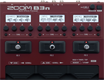# Factory : CLmelody

Discussion in 'Zoom B3n' started by Tonelib, May 20, 2018.

1. Factory : CLmelodyDevice: Zoom B3n
Firmware: 2.00

Name on device: CLmelody
Optimized for: Phones/Speaker

Effects chain:This setting, which is good for playing melodies, combines DYN Comp, D.I Plus, BassGEQ and Delay.

Effect: "DYN Comp" (Dynamics), active - "yes"
"Sense" = 8
"Attack" = Fast
"Tone" = 41
"Volume" = 41

Effect: "D.I Plus" (Overdrive / Distortion), active - "yes"
"Bass" = 52
"Middle" = 64
"Treble" = 37
"Color" = On
"Cl Volume" = 69
"Blend" = 46
"Gain" = 68
"Ds Volume" = 60

Effect: "BassGEQ" (Filter), active - "yes"
"50Hz" = 0.0
"120Hz" = 0.0
"400Hz" = 0.0
"500Hz" = 0.0
"800Hz" = -1.5
"4.5kHz" = 7.5
"10kHz" = 4.5
"Volume" = 80
"50Hz" = 0.0
"120Hz" = 0.0
"400Hz" = 0.0
"500Hz" = 2.0
"800Hz" = 4.0
"4.5kHz" = 0.0
"10kHz" = 2.0
"Volume" = 77
"Parameter set" = A

Effect: "Delay" (Delay), active - "yes"
"Time" = 486
"F.B." = 18
"Mix" = 24
"Tail" = Off

Patch Volume: 85